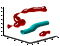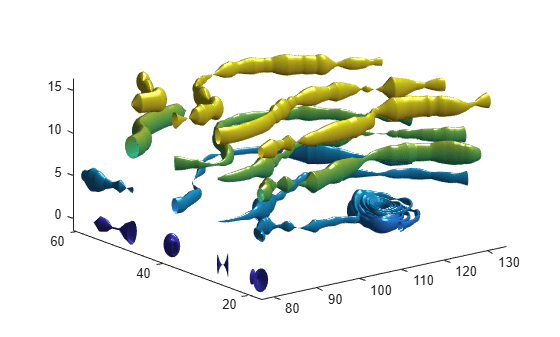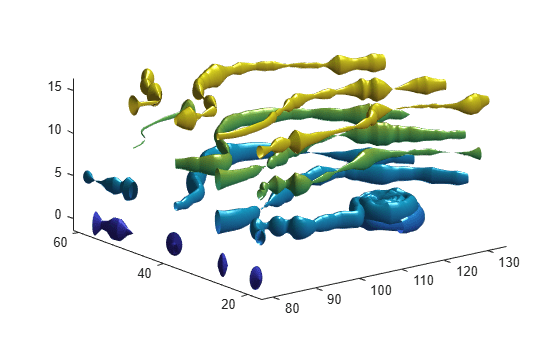# streamtube

Create 3-D stream tube plot

•## Syntax

```streamtube(X,Y,Z,U,V,W,startx,starty,startz) streamtube(U,V,W,startx,starty,startz) streamtube(vertices,X,Y,Z,divergence) streamtube(vertices,divergence) streamtube(vertices,width) streamtube(vertices) streamtube(...,[scale n]) streamtube(ax,...) h = streamtube(...) ```

## Description

`streamtube(X,Y,Z,U,V,W,startx,starty,startz)` draws stream tubes from vector volume data `U`, `V`, `W`.

The arrays `X`, `Y`, and `Z`, which define the coordinates for `U`, `V`, and `W`, must be monotonic, but do not need to be uniformly spaced. `X`, `Y`, and `Z` must have the same number of elements, as if produced by `meshgrid`.

`startx`, `starty`, and `startz` define the starting positions of the streamlines at the center of the tubes.

The width of the tubes is proportional to the normalized divergence of the vector field.

`streamtube(U,V,W,startx,starty,startz)` assumes `X`, `Y`, and `Z` are determined by the expression

```[X,Y,Z] = meshgrid(1:n,1:m,1:p) ```

where `[m,n,p] = size(U)`.

`streamtube(vertices,X,Y,Z,divergence)` assumes precomputed streamline vertices and divergence. `vertices` is a cell array of streamline vertices (as produced by `stream3`). `X`, `Y`, `Z`, and `divergence` are 3-D arrays.

`streamtube(vertices,divergence)` assumes `X`, `Y`, and `Z` are determined by the expression

```[X,Y,Z] = meshgrid(1:n,1:m,1:p) ```

where `[m,n,p] = size(divergence)`.

`streamtube(vertices,width)` specifies the width of the tubes in the cell array of vectors, `width`. The size of each corresponding element of `vertices` and `width` must be equal. `width` can also be a scalar, specifying a single value for the width of all stream tubes.

`streamtube(vertices)` selects the width automatically.

`streamtube(...,[scale n])` scales the width of the tubes by `scale`. The default is `scale = 1`. When the stream tubes are created, using start points or divergence, specifying `scale = 0` suppresses automatic scaling. `n` is the number of points along the circumference of the tube. The default is `n = 20`.

`streamtube(ax,...)` plots into the axes object `ax` instead of into the current axes object (`gca`).

`h = streamtube(...)` returns a vector of `surface` objects (one per start point) used to draw the stream tubes.

## Examples

collapse all

Use the `streamtube` function to indicate flow in the `wind` data set. The inputs include the coordinates, vector field components, and starting location for the stream tubes.

```load wind [sx,sy,sz] = meshgrid(80,20:10:50,0:5:15); streamtube(x,y,z,u,v,w,sx,sy,sz); view(3); axis tight shading interp; camlight; lighting gouraud```Use vertex data returned by the `stream3` function and divergence data to visualize flow.

```load wind [sx,sy,sz] = meshgrid(80,20:10:50,0:5:15); verts = stream3(x,y,z,u,v,w,sx,sy,sz); div = divergence(x,y,z,u,v,w); streamtube(verts,x,y,z,-div); view(3); axis tight shading interp camlight lighting gouraud```## Version History

Introduced before R2006a